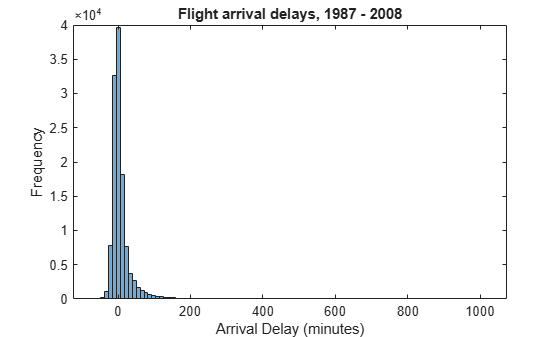Documentation

## Histograms of Tall Arrays

This example shows how to use `histogram` and `histogram2` to analyze and visualize data contained in a tall array.

### Create Tall Table

Create a datastore using the `airlinesmall.csv` data set. Treat `'NA'` values as missing data so that they are replaced with `NaN` values. Select a subset of the variables to work with. Convert the datastore into a tall table.

```varnames = {'ArrDelay', 'DepDelay', 'Year', 'Month'}; ds = datastore('airlinesmall.csv', 'TreatAsMissing', 'NA', ... 'SelectedVariableNames', varnames); T = tall(ds)```
```T = Mx4 tall table ArrDelay DepDelay Year Month ________ ________ ____ _____ 8 12 1987 10 8 1 1987 10 21 20 1987 10 13 12 1987 10 4 -1 1987 10 59 63 1987 10 3 -2 1987 10 11 -1 1987 10 : : : : : : : : ```

### Plot Histogram of Arrival Delays

Plot a histogram of the `ArrDelay` variable to examine the frequency distribution of arrival delays.

`h = histogram(T.ArrDelay);`
```Evaluating tall expression using the Local MATLAB Session: - Pass 1 of 2: Completed in 1.3 sec - Pass 2 of 2: Completed in 0.54 sec Evaluation completed in 2.8 sec ```
```title('Flight arrival delays, 1987 - 2008') xlabel('Arrival Delay (minutes)') ylabel('Frequency')```The arrival delay is most frequently a small number near 0, so these values dominate the plot and make it difficult to see other details.

### Adjust Bin Limits of Histogram

Restrict the histogram bin limits to plot only arrival delays between -50 and 150 minutes. After you create a histogram object from a tall array, you cannot change any properties that would require recomputing the bins, including `BinWidth` and `BinLimits`. Also, you cannot use `morebins` or `fewerbins` to adjust the number of bins. In these cases, use `histogram` to reconstruct the histogram from the raw data in the tall array.

```figure histogram(T.ArrDelay,'BinLimits',[-50,150])```
```Evaluating tall expression using the Local MATLAB Session: - Pass 1 of 2: Completed in 0.64 sec - Pass 2 of 2: Completed in 0.4 sec Evaluation completed in 1.4 sec ```
```title('Flight arrival delays between -50 and 150 minutes, 1987 - 2008') xlabel('Arrival Delay (minutes)') ylabel('Probability')```From this plot, it appears that long delays might be more common than initially expected. To investigate further, find the probability of an arrival delay that is one hour or greater.

### Probability of Delays One Hour or Greater

The original histogram returned an object `h` that contains the bin values in the `Values` property and the bin edges in the `BinEdges` property. You can use these properties to perform in-memory calculations.

Determine which bins contain arrival delays of one hour (60 minutes) or more. Remove the last bin edge from the logical index vector so that it is the same length as the vector of bin values.

```idx = h.BinEdges >= 60; idx(end) = [];```

Use `idx` to retrieve the value associated with each selected bin. Add the bin values together, divide by the total number of samples, and multiply by 100 to determine the overall probability of a delay greater than or equal to one hour. Since the total number of samples is computed from the original data set, use `gather` to explicitly evaluate the calculation and return an in-memory scalar.

```N = numel(T.ArrDelay); P = gather(sum(h.Values(idx))*100/N)```
```Evaluating tall expression using the Local MATLAB Session: - Pass 1 of 1: Completed in 0.35 sec Evaluation completed in 0.41 sec ```
```P = 4.4809 ```

Overall, the odds of an arrival delay one hour or longer are about 4.5%.

### Plot Bivariate Histogram of Delays by Month

Plot a bivariate histogram of the arrival delays that are 60 minutes or longer by month. This plot examines how seasonality affects arrival delay.

```figure h2 = histogram2(T.Month,T.ArrDelay,[12 50],'YBinLimits',[60 1100],... 'Normalization','probability','FaceColor','flat');```
```Evaluating tall expression using the Local MATLAB Session: - Pass 1 of 1: Completed in 1.3 sec Evaluation completed in 1.4 sec Evaluating tall expression using the Local MATLAB Session: - Pass 1 of 1: Completed in 0.85 sec Evaluation completed in 0.91 sec ```
```title('Probability of arrival delays 1 hour or greater (by month)') xlabel('Month (1-12)') ylabel('Arrival Delay (minutes)') zlabel('Probability') xticks(1:12) view(-126,23)```### Delay Statistics by Month

Use the bivariate histogram object to calculate the probability of having an arrival delay one hour or greater in each month, and the mean arrival delay for each month. Put the results in a table with the variable `P` containing the probability information and the variable `MeanByMonth` containing the mean arrival delay.

```monthNames = {'Jan','Feb','Mar','Apr','May','Jun',... 'Jul','Aug','Sep','Oct','Nov','Dec'}'; G = findgroups(T.Month); M = splitapply(@(x) mean(x,'omitnan'),T.ArrDelay,G); delayByMonth = table(monthNames, sum(h2.Values,2)*100, gather(M), ... 'VariableNames',{'Month','P','MeanByMonth'})```
```Evaluating tall expression using the Local MATLAB Session: - Pass 1 of 2: Completed in 0.51 sec - Pass 2 of 2: Completed in 1.7 sec Evaluation completed in 3.4 sec ```
```delayByMonth=12×3 table Month P MeanByMonth _______ ______ ___________ {'Jan'} 9.6497 8.5954 {'Feb'} 7.7058 7.3275 {'Mar'} 9.0543 7.5536 {'Apr'} 7.2504 6.0081 {'May'} 7.4256 5.2949 {'Jun'} 10.35 10.264 {'Jul'} 10.228 8.7797 {'Aug'} 8.5989 7.4522 {'Sep'} 5.4116 3.6308 {'Oct'} 6.042 4.6059 {'Nov'} 6.9002 5.2835 {'Dec'} 11.384 10.571 ```

The results indicate that flights in the holiday month of December have an 11.4% chance of being delayed longer than an hour, but are delayed by 10.5 minutes on average. This is closely followed by the summer months of June and July, where there is about a 10% chance of being delayed an hour or more and the average delay is roughly 9 or 10 minutes.